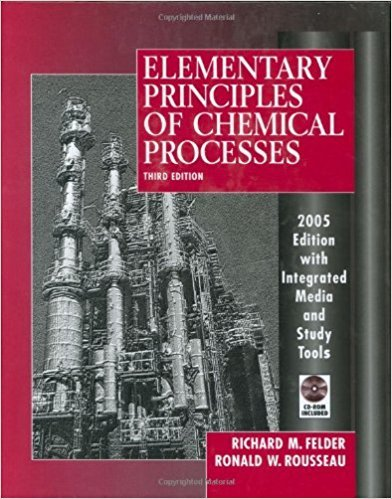×
Get Full Access to Elementary Principles Of Chemical Processes - 3 Edition - Chapter 11 - Problem 11.18
Get Full Access to Elementary Principles Of Chemical Processes - 3 Edition - Chapter 11 - Problem 11.18

×

# A gas that contains COz is contacted with liquid water inISBN: 9780471687573 143

## Solution for problem 11.18 Chapter 11

Elementary Principles of Chemical Processes | 3rd Edition

• Textbook Solutions
• 2901 Step-by-step solutions solved by professors and subject experts
• Get 24/7 help from StudySoup virtual teaching assistantsElementary Principles of Chemical Processes | 3rd Edition

4 5 1 386 Reviews
19
0
Problem 11.18

A gas that contains COz is contacted with liquid water in an agitated batch absorber. The equilibrium solubility of COz in water is given by Henry's law (Section 6.4b) CA = PA/HA where c",(mol/cmJ ) = concentration of CO2 in solution PA(atm) = partial pressure of CO2 in the gas phase HA[atm/(mol/cmJ )] = Henry's law constant The rate of absorption of CO2 (i.e., the rate of transfer of COz from the gas to the liquid per unit area of gas-liquid interface) is given by the expression rA[mol/(cmzs)] = k(C~ - CA) where CA = actual concentration of CO2 in the liquid C~ = concentration of CO2 that would be in equilibrium with the COz in the gas phase (C~ = pAlHA ) k(cm/s) = a mass transfer coefficient The gas phase is at a total pressure P(atm) and contains YA(mol COzlmol gas), and the liquid phase initially consists of V (cm3) of pure water. The agitation of the liquid phase is sufficient for its composition to be considered spatially uniform, and the amount of COz absorbed is low enough for P, V, and YA to be considered constant throughout the process. (a) Write a differential balance on COz in the liquid phase and integrate it to derive the relation CA(t) = C~[1 - exp(-kSt/V)] where S(cm2 ) is the effective contact area between the gas and liquid phases. (b) Suppose the system pressure is 20.0 atm, the liquid volume is 5.00 liters, the tank diameter is 10.0 em, the gas contains 30.0 mole% CO2, the Henry's law constant is 9230 atm/(mole/cm3 ), and the mass transfer coefficient is 0.020 cm/s. Calculate the time required for CA to reach 0.620 moUL if the gas-phase properties remain essentially constant.

Step-by-Step Solution:
Step 1 of 3

Step 2 of 3

Step 3 of 3

##### ISBN: 9780471687573

The answer to “A gas that contains COz is contacted with liquid water in an agitated batch absorber. The equilibrium solubility of COz in water is given by Henry's law (Section 6.4b) CA = PA/HA where c",(mol/cmJ ) = concentration of CO2 in solution PA(atm) = partial pressure of CO2 in the gas phase HA[atm/(mol/cmJ )] = Henry's law constant The rate of absorption of CO2 (i.e., the rate of transfer of COz from the gas to the liquid per unit area of gas-liquid interface) is given by the expression rA[mol/(cmzs)] = k(C~ - CA) where CA = actual concentration of CO2 in the liquid C~ = concentration of CO2 that would be in equilibrium with the COz in the gas phase (C~ = pAlHA ) k(cm/s) = a mass transfer coefficient The gas phase is at a total pressure P(atm) and contains YA(mol COzlmol gas), and the liquid phase initially consists of V (cm3) of pure water. The agitation of the liquid phase is sufficient for its composition to be considered spatially uniform, and the amount of COz absorbed is low enough for P, V, and YA to be considered constant throughout the process. (a) Write a differential balance on COz in the liquid phase and integrate it to derive the relation CA(t) = C~[1 - exp(-kSt/V)] where S(cm2 ) is the effective contact area between the gas and liquid phases. (b) Suppose the system pressure is 20.0 atm, the liquid volume is 5.00 liters, the tank diameter is 10.0 em, the gas contains 30.0 mole% CO2, the Henry's law constant is 9230 atm/(mole/cm3 ), and the mass transfer coefficient is 0.020 cm/s. Calculate the time required for CA to reach 0.620 moUL if the gas-phase properties remain essentially constant.” is broken down into a number of easy to follow steps, and 288 words. Elementary Principles of Chemical Processes was written by and is associated to the ISBN: 9780471687573. This full solution covers the following key subjects: gas, liquid, phase, coz, atm. This expansive textbook survival guide covers 13 chapters, and 710 solutions. Since the solution to 11.18 from 11 chapter was answered, more than 295 students have viewed the full step-by-step answer. This textbook survival guide was created for the textbook: Elementary Principles of Chemical Processes, edition: 3. The full step-by-step solution to problem: 11.18 from chapter: 11 was answered by , our top Chemistry solution expert on 11/15/17, 02:42PM.

Unlock Textbook Solution